PRINTABLE FOR KIDS

XII (12) HSC

XI (11) FYJC
X (10) SSC

# Question 1.The number √2 is shown on a number line. Steps are given to show √3 on the number line using √2. Fill in the boxes properly and complete the activity.Activity :● The point Q on the number line shows the number.......● A line perpendicular to the number line is drawn through the point Q.Point R is at unit distance from Q on the line.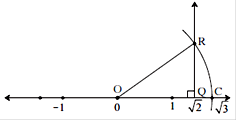● Right angled ∆ORQ is obtained by drawing seg OR.● l(OQ) = √2, l(QR) = 1∴ by Pythagoras theorem,[l(OR)]2= [l(OQ)]2+ [l(QR)]2=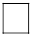2+2=+=∴ l(OR) =Draw an arc with center O and radius OR. Mark the point of intersection of the line and the arc as C. The point C shows the number √3.Answer:Activity :● The point Q on the number line shows the number ...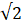... .● A line perpendicular to the number line is drawn through the point Q.Point R is at unit distance from Q on the line. (Here unit distance means 1 cm or any other unit that you choose earlier)● Right angled ∆ORQ is obtained by drawing seg OR.● l(OQ) = √2, l(QR) = 1∴ by Pythagoras theorem,[l(OR)]2= [l(OQ)]2+ [l(QR)]2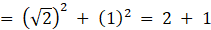= 3 ∴ l(OR) =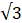The solution for drawing:To represent √3 on the number line, first of all, we have to represent √2 on the number line. The procedure for the representation of √2 will be same as shown in the activity. So, let’s start from there only. The steps further followed will be as:Step I: Now we need to construct a line which is perpendicular to line AB from point A such that this new line has unity length and let’s name the new line as AE.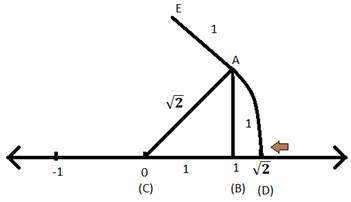Step II: Now join (C) and (E). The length of line CE could be found out by using Pythagoras theorem in right angled triangle EAC. So;AE2+ AC2= EC2⟹ EC2= 12+ (√2)2⟹ EC2= 1 + 2⟹ EC2= 3⟹ EC = √3So the length of EC line is found to be √3 units.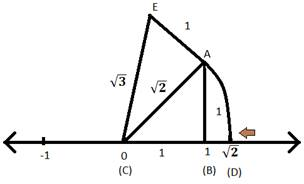Step III: Now, with (C) as center and EC as the radius of circle cut an arc on the number line and mark the point as F. Since, OE is the radius of the arc, hence OF will also be the radius of the arc and will have the same length as that of OE. So, OF = √3 units. Hence, F will represent √3 on the number line.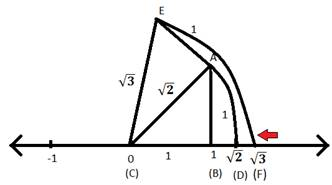Similarly, we can represent any rational number on the number line. The positive rational numbers will be represented on the right of (C) and the negative rational numbers will be on the left of (C). If m is a rational number greater than the rational number y then on the number line the point representing x will be on the right of the point represents.Question 2.Represent √5 on the number line.Answer:Steps involved are as follows:Step I: Draw a number line and mark the center point as zero.Step II: Mark right side of the zero as (1) and the left side as (-1).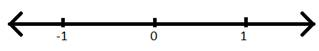Step III: We won’t be considering (-1) for our purpose.Step IV: With 2 units as length draw a line from (1) such that it is perpendicular to the line.Step V: Now join the point (0) and the end of the new line of 2 units length.Step VI: A right-angled triangle is constructed.Step VII: Now let us name the triangle as ABC such that AB is the height (perpendicular), BC is the base of triangle and AC is the hypotenuse of the right-angled ΔABC.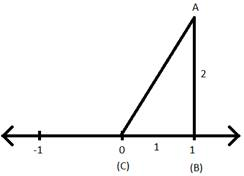Step VIII: Now the length of the hypotenuse, i.e., AC can be found by applying Pythagoras theorem to the triangle ABC.AC2= AB2+ BC2⟹ AC2= 22+ 12⟹ AC2= 4 + 1⟹ AC2= 5⟹ AC= √5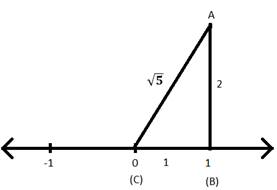Step IX: Now with AC as radius and C as the center cut an arc on the same number line and name the point as D.Step X: Since AC is the radius of the arc and hence, the CD will also be the radius of the arc whose length is √5.Step XI: Hence, D is the representation of√5 on the number line.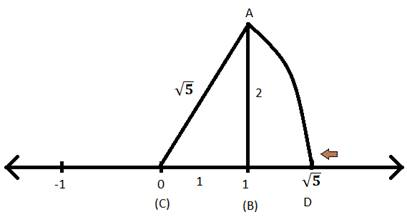Question 3.Show the number √7 on the number line.Answer:Draw a number line l and mark the points O,A and B such that OA = OB = 1. Draw BC perpendicular to number line such that BC = 1 units. Join OCIn Right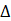OBC,OC2= OB2+ BC2= (2)2+ (1)2= 5OC =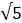Taking O as center and C and C as radius, draw an arc which cuts l in D.Hence, OC = OD =Now, draw DE perpendicular number line l such that DE = 1 Units. Join OE.In Right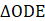,OE2= OD2+ DE2= ()2+ (1)2=5 + 1=6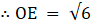Taking O as center and OE as radius, draw an arc which cuts l in F.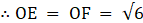Now, Draw GF perpendicular l such that GH = 1 units. Join OG.In right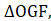OG2= OF2+ GF2= (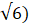2+ (1)2= 6 + 1= 7OG =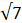Taking O as center and OG as radius, Draw an arc which cuts l in H.Hence,OG = OH =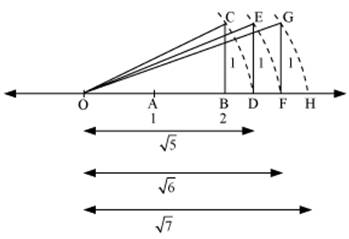## PDF FILE TO YOUR EMAIL IMMEDIATELY PURCHASE NOTES & PAPER SOLUTION. @ Rs. 50/- each (GST extra)

SUBJECTS

HINDI ENTIRE PAPER SOLUTION

MARATHI PAPER SOLUTION
SSC MATHS I PAPER SOLUTION
SSC MATHS II PAPER SOLUTION
SSC SCIENCE I PAPER SOLUTION
SSC SCIENCE II PAPER SOLUTION
SSC ENGLISH PAPER SOLUTION
SSC & HSC ENGLISH WRITING SKILL
HSC ACCOUNTS NOTES
HSC OCM NOTES
HSC ECONOMICS NOTES
HSC SECRETARIAL PRACTICE NOTES

2019 Board Paper Solution

HSC ENGLISH SET A 2019 21st February, 2019

HSC ENGLISH SET B 2019 21st February, 2019

HSC ENGLISH SET C 2019 21st February, 2019

HSC ENGLISH SET D 2019 21st February, 2019

SECRETARIAL PRACTICE (S.P) 2019 25th February, 2019

HSC XII PHYSICS 2019 25th February, 2019

CHEMISTRY XII HSC SOLUTION 27th, February, 2019

OCM PAPER SOLUTION 2019 27th, February, 2019

HSC MATHS PAPER SOLUTION COMMERCE, 2nd March, 2019

HSC MATHS PAPER SOLUTION SCIENCE 2nd, March, 2019

SSC ENGLISH STD 10 5TH MARCH, 2019.

HSC XII ACCOUNTS 2019 6th March, 2019

HSC XII BIOLOGY 2019 6TH March, 2019

HSC XII ECONOMICS 9Th March 2019

SSC Maths I March 2019 Solution 10th Standard11th, March, 2019

SSC MATHS II MARCH 2019 SOLUTION 10TH STD.13th March, 2019

SSC SCIENCE I MARCH 2019 SOLUTION 10TH STD. 15th March, 2019.

SSC SCIENCE II MARCH 2019 SOLUTION 10TH STD. 18th March, 2019.

SSC SOCIAL SCIENCE I MARCH 2019 SOLUTION20th March, 2019

SSC SOCIAL SCIENCE II MARCH 2019 SOLUTION, 22nd March, 2019

XII CBSE - BOARD - MARCH - 2019 ENGLISH - QP + SOLUTIONS, 2nd March, 2019

HSC Maharashtra Board Papers 2020

(Std 12th English Medium)

HSC ECONOMICS MARCH 2020

HSC OCM MARCH 2020

HSC ACCOUNTS MARCH 2020

HSC S.P. MARCH 2020

HSC ENGLISH MARCH 2020

HSC HINDI MARCH 2020

HSC MARATHI MARCH 2020

HSC MATHS MARCH 2020

SSC Maharashtra Board Papers 2020

(Std 10th English Medium)

English MARCH 2020

HindI MARCH 2020

Hindi (Composite) MARCH 2020

Marathi MARCH 2020

Mathematics (Paper 1) MARCH 2020

Mathematics (Paper 2) MARCH 2020

Sanskrit MARCH 2020

Important-formula

THANKS